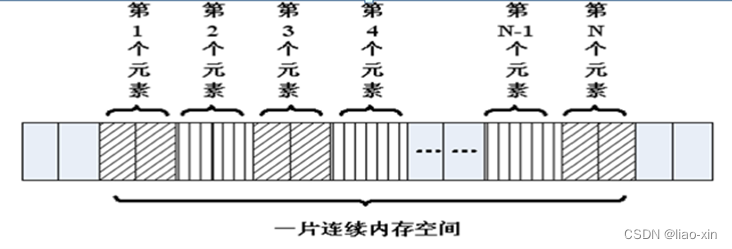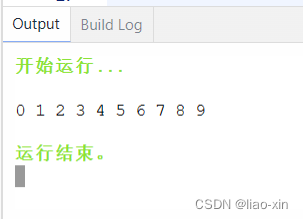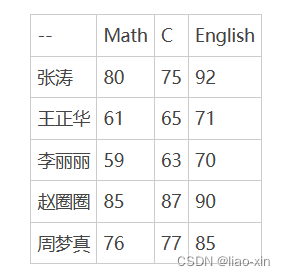# C语言 —— 数组

### 一维数组与二维数组

• 前言
• 一、 一维数组
• 1.1 一维数组定义和使用
• 1.2 例子 ：列出0-9的数字
• 1.3 一维数组的初始化
• 1.4 数组名
• 二、二维数组
• 2.1 二维数组的定义
• 2.2 例题：一个学习小组有 5 个人，每个人有 3 门课程的考试成绩，求该小组各科的平均分和总平均分。
• 2.3 二维数组初始化
• 总结

# 前言# 一、 一维数组

## 1.1 一维数组定义和使用

1、数组名字符合标识符的书写规定(数字、英文字母、下划线)。
2、数组名不能与其它变量名相同，同一作用域内是唯一的。
3、方括号 [ ] 中常量表达式表示数组元素的个数。

int a表示数组a有3个元素

## 1.2 例子 ：列出0-9的数字

``````#include <stdio.h>
int main()
{
int a;//定义了一个数组，名字叫a，有10个成员，每个成员都是int类型
//a…… a，没有a
//没有a这个变量，a是数组的名字，但不是变量名，它是常量
a = 0;
//……
a = 9;
int i = 0;
for (i = 0; i < 10; i++)
{
a[i] = i; //给数组赋值
}

//遍历数组，并输出每个成员的值
for (i = 0; i < 10; i++)
{
printf("%d ", a[i]);
}
printf("\n");
return 0;
}
``````## 1.3 一维数组的初始化

1. 可以只给部分元素赋值。当{ }中值的个数少于元素个数时，只给前面部分元素赋值。
2. 只能给元素逐个赋值，不能给数组整体赋值
3. 如给全部元素赋值，那么在定义数组时可以不给出数组长度
代码例子：
``````int a = { 1, 2, 3, 4, 5, 6, 7, 8, 9, 10 };//定义一个数组，同时初始化所有成员变量
int a = { 1, 2, 3 };//初始化前三个成员，后面所有元素都设置为0
int a = { 0 };//所有的成员都设置为0
//[]中不定义元素个数，定义时必须初始化
int a[] = { 1, 2, 3, 4, 5 };//定义了一个数组，有5个成员
``````

## 1.4 数组名

``````#include <stdio.h>

int main()
{
int a = { 1, 2, 3, 4, 5, 6, 7, 8, 9, 10 };//定义一个数组，同时初始化所有成员变量
printf("a = %p\n", a);
printf("&a = %p\n", &a);
int n = sizeof(a); //数组占用内存的大小，10个int类型，10 * 4  = 40
int n0 = sizeof(a);//数组第0个元素占用内存大小，第0个元素为int，4
int i = 0;
for (i = 0; i < sizeof(a) / sizeof(a); i++)
{
printf("%d ", a[i]);
}
printf("\n");
return 0;
}

``````

# 二、二维数组

## 2.1 二维数组的定义

``````dataType arrayName[length1][length2];
``````

``````int a;
``````

``````a, a, a, a
a, a, a, a
a, a, a, a
``````

## 2.2 例题：一个学习小组有 5 个人，每个人有 3 门课程的考试成绩，求该小组各科的平均分和总平均分。``````#include <stdio.h>
int main(){
int i, j;  //二维数组下标
int sum = 0;  //当前科目的总成绩
int average;  //总平均分
int v;  //各科平均分
int a;  //用来保存每个同学各科成绩的二维数组
printf("Input score:\n");
for(i=0; i<3; i++){
for(j=0; j<5; j++){
scanf("%d", &a[j][i]);  //输入每个同学的各科成绩
sum += a[j][i];  //计算当前科目的总成绩
}
v[i]=sum/5;  // 当前科目的平均分
sum=0;
}
average = (v + v + v) / 3;
printf("Math: %d\nC Languag: %d\nEnglish: %d\n", v, v, v);
printf("Total: %d\n", average);
return 0;
}
``````

## 2.3 二维数组初始化

1. 可以只对部分元素赋值，未赋值的元素自动取“零”值。
2. 如果对全部元素赋值，那么第一维的长度可以不给出。
3. 二维数组可以看作是由一维数组嵌套而成的；如果一个数组的每个元素又是一个数组，那么它就是二维数组。